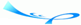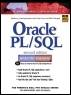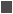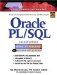# Lab 11.1 RAISE_APPLICATION_ERROR

 Team-FlyOracle® PL/SQL® Interactive Workbook, Second EditionBy Benjamin Rosenzweig, Elena Silvestrova Table of Contents Chapter 11.  Exceptions: Advanced Concepts

Lab Objective

After this Lab, you will be able to:

• Use RAISE_APPLICATION_ERROR

RAISE_APPLICATION_ERROR is a special built-in procedure provided by Oracle. This procedure allows programmers to create meaningful error messages for a specific application. The RAISE_APLICATION_ERROR procedure works with user-defined exceptions. The syntax of the RAISE_APPLICATION_ERROR is

` RAISE_APPLICATION_ERROR(error_number, error_message); `

or

` RAISE_APPLICATION_ERROR(error_number, error_message,     keep_errors); `

As you can see, there are two forms of the RAISE_APPLICATION_ERROR procedure. The first form contains only two parameters: error_number and error_ message. The error_number is a number of the error that a programmer associates with a specific error message, and can be any number between -20,999 and -20,000. The error_message is the text of the error, and it can contain up to 512 characters.

The second form of RAISE_APPLICATION_ERROR contains one additional parameter: keep_errors. Keep_errors is an optional Boolean parameter. If keep_errors is set to TRUE, the new error will be added to the list of errors that has been raised already. If keep_errors is set to FALSE, the new error replaces the list of errors that has been raised already. The default value for the parameter keep_errors is FALSE.

It is important for you to note that the RAISE_APPLICATION_ERROR procedure works with unnamed user-defined exceptions. It associates the number of the error with the text of the error. Therefore, the user-defined exception does not have a name associated with it.

Consider the following example used in Chapter 10. This example illustrates the use of the named user-defined exception and the RAISE statement. Within the example you will be able to compare a modified version using the unnamed user-defined exception and the RAISE_APPLICATION_ERROR procedure.FOR EXAMPLE

First, view the original example from Chapter 10. Notice that the named user-defined exception and the RAISE statement are shown in bold letters.

` DECLARE     v_student_id STUDENT.STUDENT_ID%TYPE := &sv_student_id;     v_total_courses NUMBER;     e_invalid_id EXCEPTION;  BEGIN     IF v_student_id < 0 THEN        RAISE e_invalid_id;     ELSE        SELECT COUNT(*)          INTO v_total_courses          FROM enrollment         WHERE student_id = v_student_id;        DBMS_OUTPUT.PUT_LINE ('The student is registered for           '||v_total_courses||' courses');     END IF;     DBMS_OUTPUT.PUT_LINE ('No exception has been raised');  EXCEPTION     WHEN e_invalid_id THEN        DBMS_OUTPUT.PUT_LINE ('An id cannot be negative');  END; `

Now, compare the modified example as follows (changes are shown in bold letters):

` DECLARE     v_student_id STUDENT.STUDENT_ID%TYPE := &sv_student_id;     v_total_courses NUMBER;  BEGIN     IF v_student_id < 0 THEN        RAISE_APPLICATION_ERROR           (-20000, 'An id cannot be negative');     ELSE        SELECT COUNT(*)          INTO v_total_courses          FROM enrollment         WHERE student_id = v_student_id;        DBMS_OUTPUT.PUT_LINE ('The student is registered for           '||v_total_courses||' courses');     END IF;  END; `

The second version of the example does not contain the name of the exception, the RAISE statement, nor the error-handling section of the PL/SQL block. Instead, it has a single RAISE_APPLICATION_ERROR statement.Even though the RAISE_APPLICATION_ERROR is a built-in procedure, it can be referred to as a statement when used in the PL/SQL block.

Both versions of the example achieve the same result: The processing stops if a negative number is provided for v_student_id. However, the second version of this example produces the output that has the look and feel of an error message. Now, run both versions of the example with the value of -4 for the variable v_student_id.

The first version of the example produces the following output:

` Enter value for sv_student_id: -4  old   2: v_student_id STUDENT.STUDENT_ID%TYPE := &sv_  student_id;  new   2: v_student_id STUDENT.STUDENT_ID%TYPE := -4;  An id cannot be negative  PL/SQL procedure successfully completed. `

The second version of the example produces the following output:

` Enter value for sv_student_id: -4  old   2:    v_student_id STUDENT.STUDENT_ID%TYPE :=  &sv_student_id;  new   2:    v_student_id STUDENT.STUDENT_ID%TYPE := -4;  DECLARE  *  ERROR at line 1:  ORA-20000: An id cannot be negative  ORA-06512: at line 6 `

The output produced by the first version of the example contains the error message "An id cannot be negative" and the message "PL/SQL completed . . .". The error message "An id cannot . . ." in the output generated by the second version of the example looks like the error message generated by the system, because the error number ORA-20000 precedes the error message.

The RAISE_APPLICATION_ERROR procedure can work with built-in exceptions as well. Consider the following example:FOR EXAMPLE

` DECLARE     v_student_id STUDENT.STUDENT_ID%TYPE := &sv_student_id;     v_name VARCHAR2(50);  BEGIN     SELECT first_name||' '||last_name       INTO v_name       FROM student      WHERE student_id = v_student_id;     DBMS_OUTPUT.PUT_LINE (v_name);  EXCEPTION     WHEN NO_DATA_FOUND THEN        RAISE_APPLICATION_ERROR (-20001, 'This ID is invalid');  END; `

When the value of 100 is entered for the student ID, the example produces the output shown:

` Enter value for sv_student_id: 100  old   2:    v_student_id STUDENT.STUDENT_ID%TYPE :=  &sv_student_id;  new   2:    v_student_id STUDENT.STUDENT_ID%TYPE := 100;  DECLARE  *  ERROR at line 1:  ORA-20001: This ID is invalid  ORA-06512: at line 12 `

The built-in exception NO_DATA_FOUND is raised because there is no record in the STUDENT table corresponding to this value of the student ID. However, the number of the error message does not refer to the exception NO_DATA_FOUND. It refers to the error message "This ID is invalid."

The RAISE_APPLICATION_ERROR procedure allows programmers to return error messages in a manner that is consistent with Oracle errors. However, it is important for you to note that it is up to a programmer to maintain the relationship between the error numbers and the error messages. For example, you have designed an application to maintain the enrollment information on students. In this application you have associated the error text "This ID is invalid" with the error number ORA-20001. This error message can be used by your application for any invalid ID. Once you have associated the error number (ORA-20001) with a specific error message (This ID is invalid), you should not assign this error number to another error message. If you do not maintain the relationship between error numbers and error messages, the error-handling interface of your application might become very confusing to the users and to yourself.

#### Lab 11.1 Exercises

##### 11.1.1 Use RAISE_APPLICATION_ERROR

In this exercise, you calculate how many students are registered for each course. You then display a message on the screen that contains the course number and the number of students registered for it. The original PL/SQL script will not contain any exception handlers, so you will be asked to add the RAISE_APPLICATION_ERROR statement.

Create the following PL/SQL script:

` -- ch11_1a.sql, version 1.0  SET SERVEROUTPUT ON  DECLARE     CURSOR course_cur IS        SELECT course_no, section_id          FROM section        ORDER BY course_no, section_id;     v_cur_course SECTION.COURSE_NO%TYPE := 0;     v_students NUMBER(3) := 0;     v_total NUMBER(3) := 0;  BEGIN     FOR course_rec IN course_cur LOOP        IF v_cur_course = 0 THEN           v_cur_course := course_rec.course_no;        END IF;        SELECT COUNT(*)          INTO v_students          FROM enrollment         WHERE section_id = course_rec.section_id;        IF v_cur_course = course_rec.course_no THEN           v_total := v_total + v_students;        ELSE           DBMS_OUTPUT.PUT_LINE ('Course '||v_cur_course||              ' has '||v_total||' student(s)');           v_cur_course := course_rec.course_no;           v_total := 0;        END IF;     END LOOP;     DBMS_OUTPUT.PUT_LINE ('Done…');  END; `

Take a closer look this script. As you learned earlier, this script determines the number of students registered for each course. It then displays the course number and the number of students on the screen. In order to achieve these results, the cursor needs to be defined on the SECTION table. This cursor retrieves the course numbers and section IDs. It also now defines three variables: v_cur_course, v_students, and v_total.

The variable v_cur_course holds the number of the current course. There are duplicate course numbers in the SECTION table, because a course can have multiple sections. In order to display the number of students for each course rather than each section, you need to store the number of the current course. For example, course 10 has three sections: 1, 2, and 3. Section 1 has 3 students, section 2 has 5 students, and section 3 has 10 students. Therefore, course 10 has 18 students. Once this number is calculated, the message "10 has 18 student(s)" can be displayed on the screen. As a result, you need to compare the variable v_cur_course to the course number returned by the cursor.

The variable v_students holds the number of students registered for a specific section of a course. As long as the value of the variable v_cur_course equals the value of the course_rec.course_no, the variable v_students is added to the current value of the variable v_total, which holds the total number of students registered for a given course.

Notice that in the body of the cursor FOR loop, there are two IF statements. The first IF statement

` IF v_cur_course = 0 THEN     v_cur_course := course_rec.course_no;  END IF; `

is executed only once, for the first iteration of the cursor FOR loop. This IF statement guarantees that the value of course_rec.course_no is assigned to the variable v_cur_course before any further processing.

The second IF statement

` IF v_cur_course = course_rec.course_no THEN     v_total := v_total + v_students;  ELSE     DBMS_OUTPUT.PUT_LINE ('Course '||v_cur_course||' has '||        v_total||' student(s)');     v_cur_course := course_rec.course_no;     v_total := 0;  END IF; `

compares the value of v_cur_course to the value of the course_rec.course_no. For the first iteration of the cursor FOR loop, this condition of the IF statement evaluates to TRUE, and the value of v_students is added to the current value of v_total. For the next iteration of the cursor FOR loop, the IF statement evaluates to TRUE if the course number has not changed. However, if the course number has changed, this IF statement evaluates to FALSE, and the ELSE part of the IF statement is executed. Therefore, the DBMS_OUTPUT.PUT_LINE statement displays the course information on the screen, the value of the course_rec.course_no is assigned to the variable v_cur_course, and the value of the variable v_total is set to 0 again. Why do you think the variable v_total must be set to 0?

Execute the script, and then answer the following questions:

 a) What output was printed on the screen? b) Modify this script so that if a course has more than 20 students enrolled in it, an error message is displayed indicating that this course has too many students enrolled. c) Execute the new version of the script. What output was printed on the screen? d) Generally, when an exception is raised and handled inside a loop, the loop does not terminate prematurely. Why do you think the cursor FOR loop terminates as soon as RAISE_ APPLICATION_ERROR executes?

#### Lab 11.1 Exercise Answers

This section gives you some suggested answers to the questions in Lab 11.1, with discussion related to how those answers resulted. The most important thing to realize is whether your answer works. You should figure out the implications of the answers here and what the effects are from any different answers you may come up with.

##### 11.1.1 Answers

 a) What output was printed on the screen? A1: ` Course 10 has 1 student(s) Course 20 has 6 student(s) Course 25 has 40 student(s) Course 100 has 7 student(s) Course 120 has 19 student(s) Course 122 has 20 student(s) Course 124 has 3 student(s) Course 125 has 6 student(s) Course 130 has 6 student(s) Course 132 has 0 student(s) Course 134 has 2 student(s) Course 135 has 2 student(s) Course 140 has 7 student(s) Course 142 has 3 student(s) Course 144 has 0 student(s) Course 145 has 0 student(s) Course 146 has 1 student(s) Course 147 has 0 student(s) Course 204 has 0 student(s) Course 210 has 0 student(s) Course 220 has 0 student(s) Course 230 has 2 student(s) Course 240 has 1 student(s) Course 310 has 0 student(s) Course 330 has 0 student(s) Course 350 has 9 student(s) Course 420 has 0 student(s) Course 430 has 0 student(s) Done… PL/SQL procedure successfully completed. `Notice that each course number is displayed a single time only. b) Modify this script so that if a course has more than 20 students enrolled in it, an error message is displayed indicating that this course has too many students enrolled. A2: Answer: Your script should look similar to the script shown. All changes are shown in bold letters.` -- ch11_1b.sql, version 2.0 SET SERVEROUTPUT ON DECLARE CURSOR course_cur IS SELECT course_no, section_id FROM section ORDER BY course_no, section_id; v_cur_course SECTION.COURSE_NO%TYPE := 0; v_students NUMBER(3) := 0; v_total NUMBER(3) := 0; BEGIN FOR course_rec IN course_cur LOOP IF v_cur_course = 0 THEN v_cur_course := course_rec.course_no; END IF; SELECT COUNT(*) INTO v_students FROM enrollment WHERE section_id = course_rec.section_id; IF v_cur_course = course_rec.course_no THEN v_total := v_total + v_students; IF v_total > 20 THEN RAISE_APPLICATION_ERROR (-20002, 'Course '|| v_cur_course||' has too many students'); END IF; ELSE DBMS_OUTPUT.PUT_LINE ('Course '||v_cur_course|| 'has '||v_total||' student(s)'); v_cur_course := course_rec.course_no; v_total := 0; END IF; END LOOP; DBMS_OUTPUT.PUT_LINE ('Done…'); END; `Consider the result if you were to add another IF statement to this script, one in which the IF statement checks whether the value of the variable exceeds 20. If the value of the variable does exceed 20, the RAISE_APPLICATION_ERROR statement executes, and the error message is displayed on the screen. c) Execute the new version of the script. What output was printed on the screen? A3: Answer: Your output should look similar to the following:` Course 10 has 1 student(s) Course 20 has 6 student(s) DECLARE * ERROR at line 1: ORA-20002: Course 25 has too many students ORA-06512: at line 21 `Course 25 has 40 students enrolled. As a result, the IF statement` IF v_total > 20 THEN RAISE_APPLICATION_ERROR (-20002, 'Course '|| v_cur_course||' has too many students'); END IF; `evaluates to TRUE, and the unnamed user-defined error is displayed on the screen. d) Generally, when an exception is raised and handled inside a loop, the loop does not terminate prematurely. Why do you think the cursor FOR loop terminates as soon as RAISE_ APPLICATION_ERROR executes? A4: Answer: When the RAISE_APPLIC ATION_ERROR procedure is used to handle a user-defined exception, control is passed to the host environment as soon as the error is handled.Therefore, the cursor FOR loop terminates prematurely. In this case, it terminates as soon as the course that has more than 20 students registered for it is encountered.When a user-defined exception is used with the RAISE statement, the exception propagates from the inner block to the outer block. For example:` -- outer block BEGIN FOR record IN cursor LOOP -- inner block BEGIN RAISE my_exception; EXCEPTION WHEN my_exception THEN DBMS_OUTPUT.PUT_LINE ('An error has occurred'); END; END LOOP; END; `In this example, the exception my_exception is raised and handled in the inner block. Control of the execution is passed to the outer block once the exception my_exception is raised. As a result, the cursor FOR loop will not terminate prematurely.When the RAISE_APPLICATION_ERROR procedure is used, control is always passed to the host environment. The exception does not propagate from the inner block to the outer block. Therefore, any loop defined in the outer block will terminate prematurely if an error has been raised in the inner block, with the help of the RAISE_APPLICATION_ERROR procedure.

#### Lab 11.1 Self-Review Questions

In order to test your progress, you should be able to answer the following questions.

Answers appear in Appendix A, Section 11.1.

 1) The RAISE_APPLICATION_ERROR works with which of the following? _____ Named user-defined exceptions only_____ Unnamed user-defined exceptions only_____ Built-in and unnamed user-defined exceptions 2) The RAISE_APPLICATION_ERROR procedure requires which of the following parameters? _____ error_number, error_text, keep_error_____ error_text, keep_error_____ error_number, error_text 3) The error number used in the RAISE_APPLICATION_ERROR must be which of the following? _____ A number between -20,000 and -20,999_____ A number between 20,000 and 20,999 4) The RAISE_APLICATION_ERROR halts the execution of the program. _____ True_____ False 5) When the parameter keep_error is set to TRUE, which of the following occurs? _____ An error message is displayed on the screen._____ An error number is displayed on the screen._____ A new error message is added to the list of raised error messages.

 Team-FlyTopOracle PL/SQL Interactive Workbook (2nd Edition)
ISBN: 0130473200
EAN: 2147483647
Year: 2002
Pages: 146

Similar book on Amazon

flylib.com © 2008-2017.
If you may any questions please contact us: flylib@qtcs.net